# NCERT Solutions for Class 9 Maths Chapter 13 - Surface Areas and Volumes

Share

The Class 9 Chapter 13 - Surface Area and Volumes NCERT Solutions have been made available here in a step-by-step manner. These Solutions have been expertly created by Goprep’s teachers who have thorough understanding of the topics discussed in the Chapter. So, if you are looking to master the various concepts explained in the Surface Areas and Volumes Chapter, you can do so by referring to these NCERT Solutions for Chapter 13.

The Class 9 Maths Chapter 13 mainly deals with the concept of Surface Areas and Volumes. Further, the Chapter goes on to explain critical topics such as the Surface Area of a Cuboid and a Cube, Surface Area of a Sphere, Volume of a Cylinder and Volume of a Sphere among others. So, to gain a better insight into all these topics and prepare the Chapter in a proper time frame, you can simply take the help of our NCERT Solutions For Class 9 Maths Chapter 13.

## NCERT Solutions for Class 9 Maths Chapter 13 - Surface Areas and Volumes

Exercise 13.1
• Exercise 13.1
• Exercise 13.2
• Exercise 13.3
• Exercise 13.4
• Exercise 13.5
• Exercise 13.6
• Exercise 13.7
• Exercise 13.8
• Exercise 13.9
Class 9th|NCERT - MathematicsChapter 13 - Surface Areas and Volumes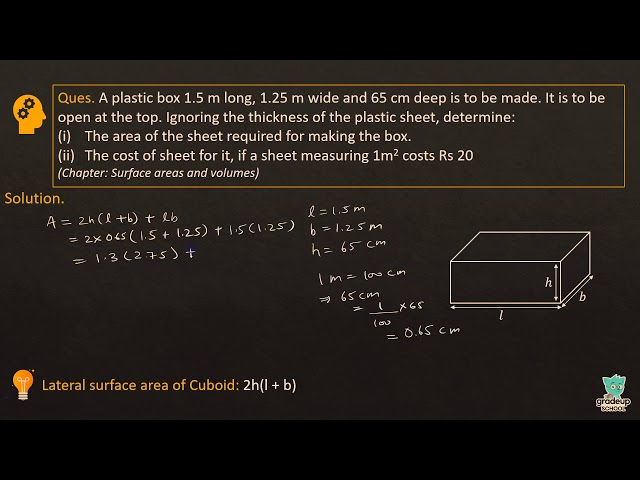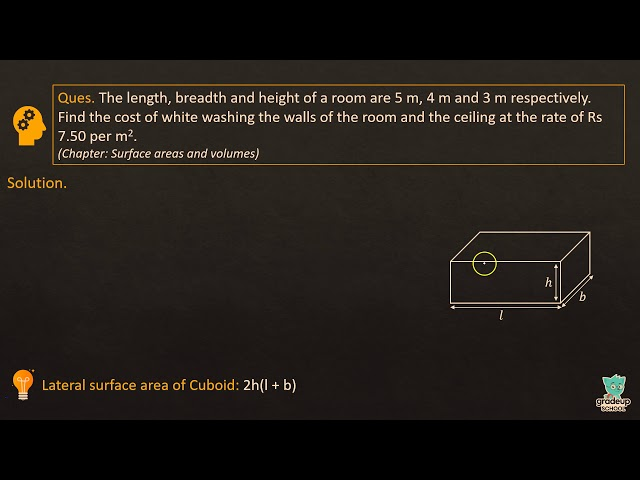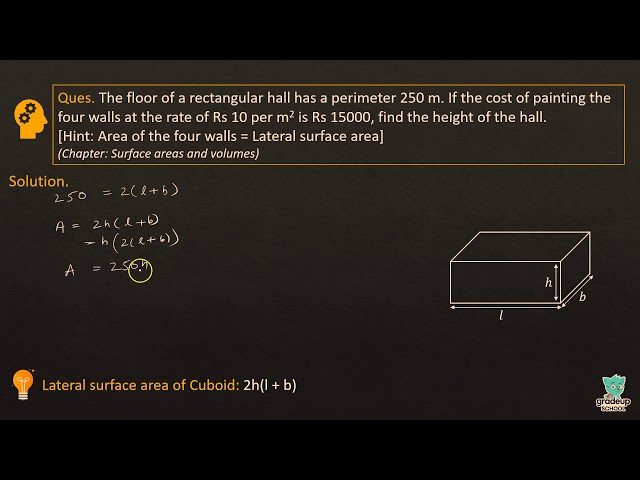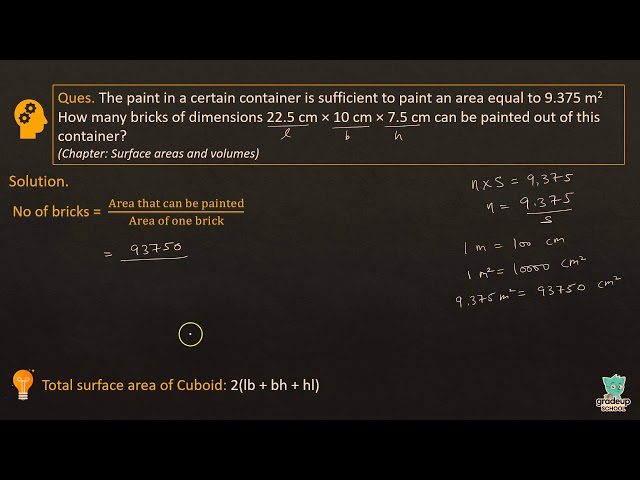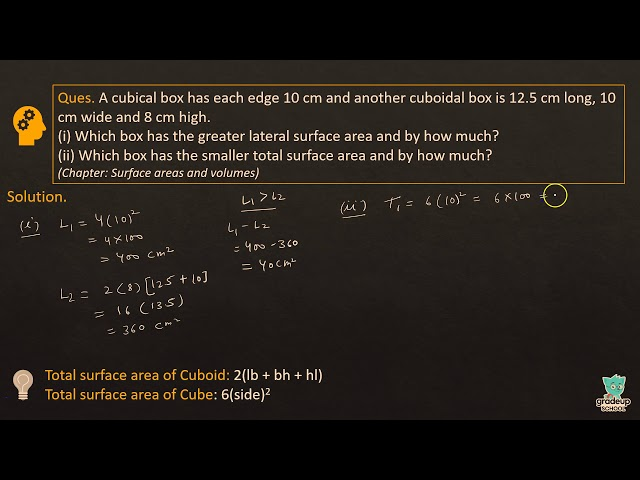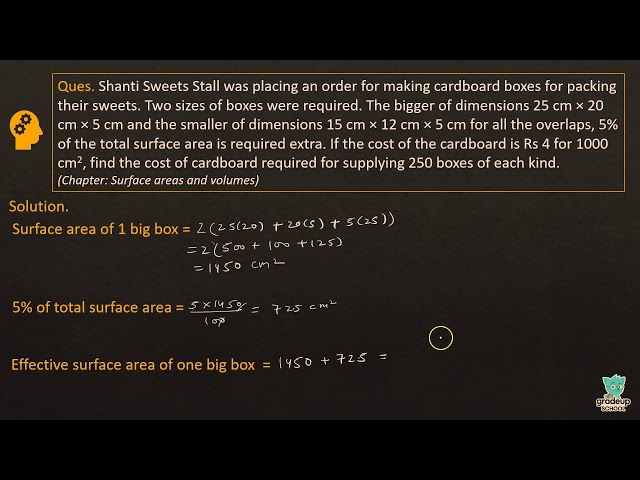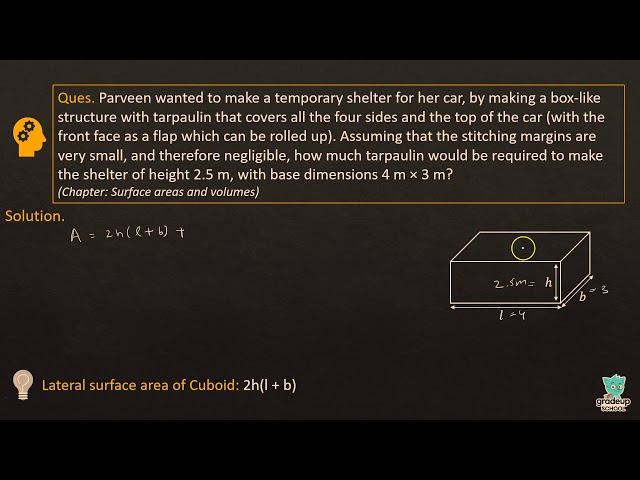NCERT - MathematicsClass 9th , NCERT
 Chapter 1 - Number System Chapter 2 - Polynomials Chapter 3 - Coordinate Geometry Chapter 4 - Linear Equations in two Variables Chapter 5 - Introduction to Euclid's Geometry Chapter 6 - Lines and Angles Chapter 7 - Triangles Chapter 8 - Quadrilaterals Chapter 9 - Areas of Parallelograms and Triangles Chapter 10 - Circles Chapter 11 - Constructions Chapter 12 - Heron's Formula Chapter 13 - Surface Areas and Volumes Chapter 14 - Statistics Chapter 15 - Probability